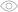# How Well Do You Know Arrays in C++?3067Arrays are often used in programming and their use in C++ language is quite extensive. This quiz includes both theoretical and practical tasks on the subject and will help detect your weak areas and highlight your skills.

What is an array?

A table storing different values

A structure of data storing a set of values of the same type united by a single name and identified using an index

A cell in the computer memory where one value can be stored

What can arrays be?

Simple and complex

Homogeneous and heterogeneous

One-dimensional and multidimensional

How to declare a one-dimensional array named array consisting of 10 integer-type elements?

First, you need to specify the array type, then name, then - the number of elements in square brackets.

int array;

array;

int array(10);

There's an array: int array = { 3, 10, 7, 9, 2}. How do you refer to number 7?

Reference to array elements is made by the index, with the counting starting from 0.

array;

array;

array;

Is this array declared correctly?

You cannot declare a statistical array having the variable value as an amount

Yes

No

What does this code fragment do?

Calculates the number of elements in the array

Calculates the sum of first 10 elements of the array

Calculates the sum of indexes of the array

What does this code do?

Creates a dynamic array from n elements

It fills the array with the entered value n

The code is wrong, so there will be an error

What is a two-dimensional array?

An array uniting two arrays

An array made of two rows

An array of one-dimensional arrays

Where is a two-dimensional array declared correctly?

The first value in square brackets indicates the number of rows, the second - columns.

An array is shown in the picture. What element will be under the name a?

Since indexing starts from 0, when referring to element a the index will be beyond the array. To refer to the element with value 8 one needs to use a, and to refer to 4 - a.

8

4

Not any

What sorting method is shown in the picture?

Bubble Sort

Insertion Sort

Merge Sort

A two-dimentional array and a code fragment are shown in the picture. What will appear in the pic as a result of the code execution?

In the code, the sum of the first three elements of the row with index 1 is calculated, this being said, the element is used only in the case that it is positive Thus, the the cycle contains elements with values -9, 8,7. Of them only 8 and 7 are positive.

6

15

3

You need to declare a two-dimensional dynamic array from 2 rows and 5 columns. How would you do it?

How would you fill an array with random integral values from 10 to 100?

To correctly fill with random values from a range you need to use the formula: rand() % ( b – a + 1 ) + a; where a – the minimal value of the range, and b – the maximal.

What is delete array[]used for?

To clear array

To clear memory under array

To delete the elements of array

You showed the initial level of knowledge on the subject

You still have a lot to know about arrays! Read theory, solve problems, learn, believe: you can do it! Share the quiz with friends and find out how many questions they can answer.

You showed the middle level of knowledge on the subject

You aren't bad at arrays but still, there are some gaps in knowledge. Read corresponding articles and textbooks, solve problems and you'll easily make up for a deficiency in your knowledge! Share the quiz with friends and find out how many questions they'll answer.

You know the subject in perfection!

You solved all problems with no trouble. Congrats: you mastered this subject in perfection. It's time to take on something harder! Share the quiz with friends and find out how many questions they'll answer.

What is an array?
1 / 15
What can arrays be?
2 / 15
How to declare a one-dimensional array named array consisting of 10 integer-type elements?
3 / 15
There's an array: int array = { 3, 10, 7, 9, 2}. How do you refer to number 7?
4 / 15
Is this array declared correctly?
5 / 15What does this code fragment do?
6 / 15What does this code do?
7 / 15What is a two-dimensional array?
8 / 15
Where is a two-dimensional array declared correctly?
9 / 15
An array is shown in the picture. What element will be under the name a?
10 / 15What sorting method is shown in the picture?
11 / 15A two-dimentional array and a code fragment are shown in the picture. What will appear in the pic as a result of the code execution?
12 / 15You need to declare a two-dimensional dynamic array from 2 rows and 5 columns. How would you do it?
13 / 15
How would you fill an array with random integral values from 10 to 100?
14 / 15
What is delete array[]used for?
15 / 15

### Calculating results...

Useful and short ads help us create new content every day.